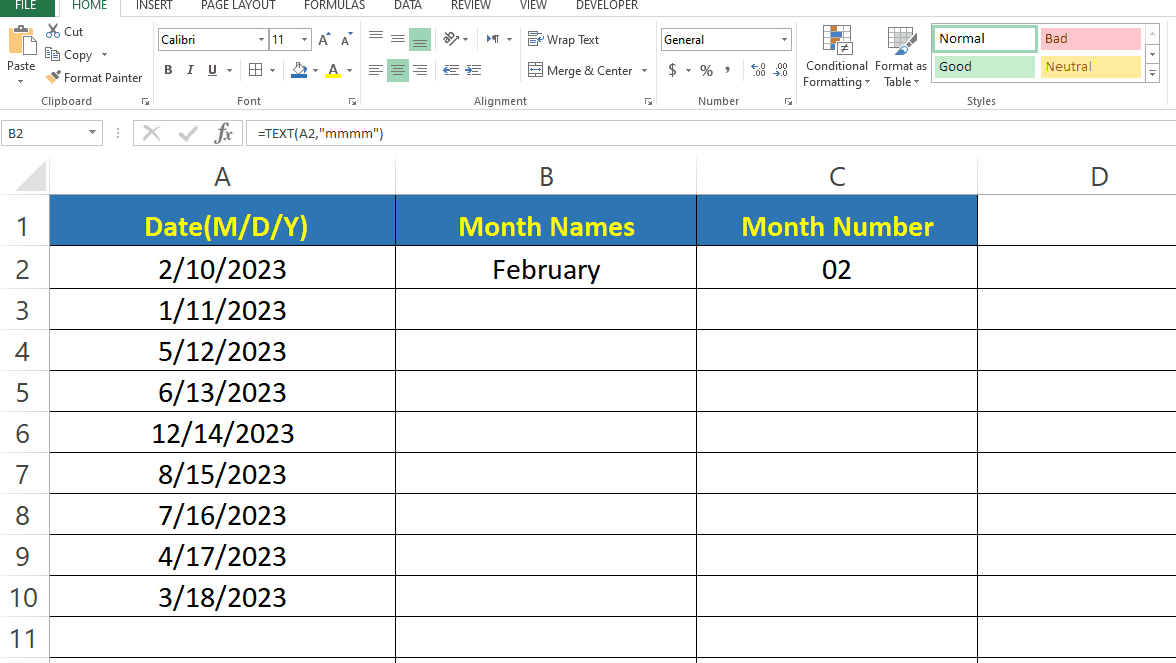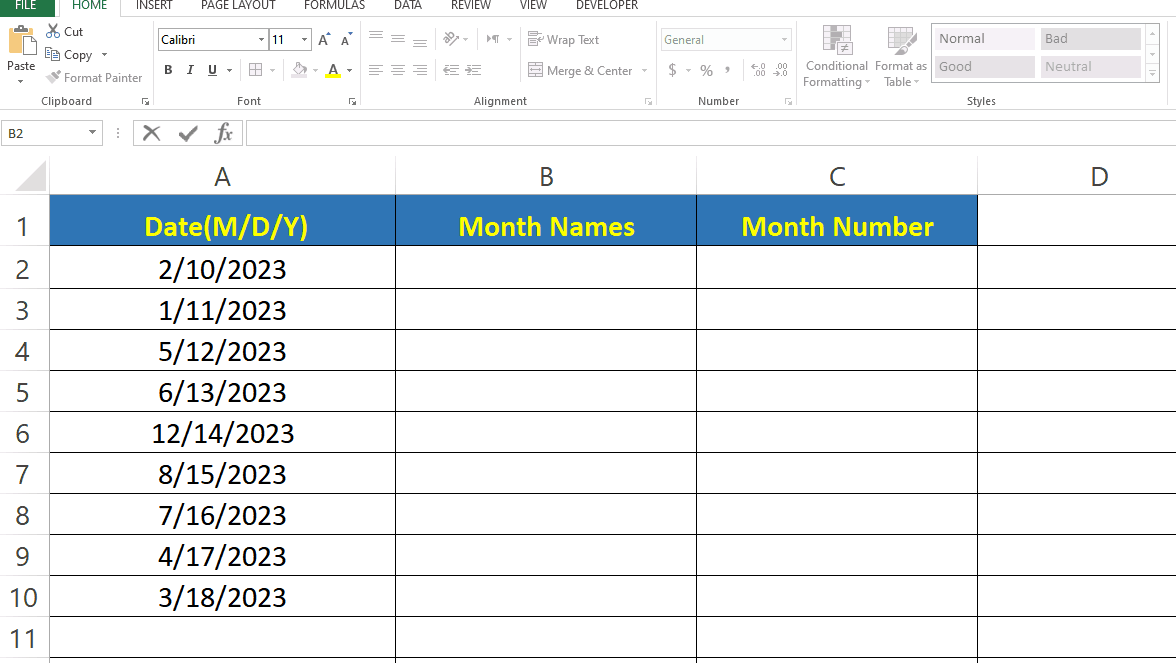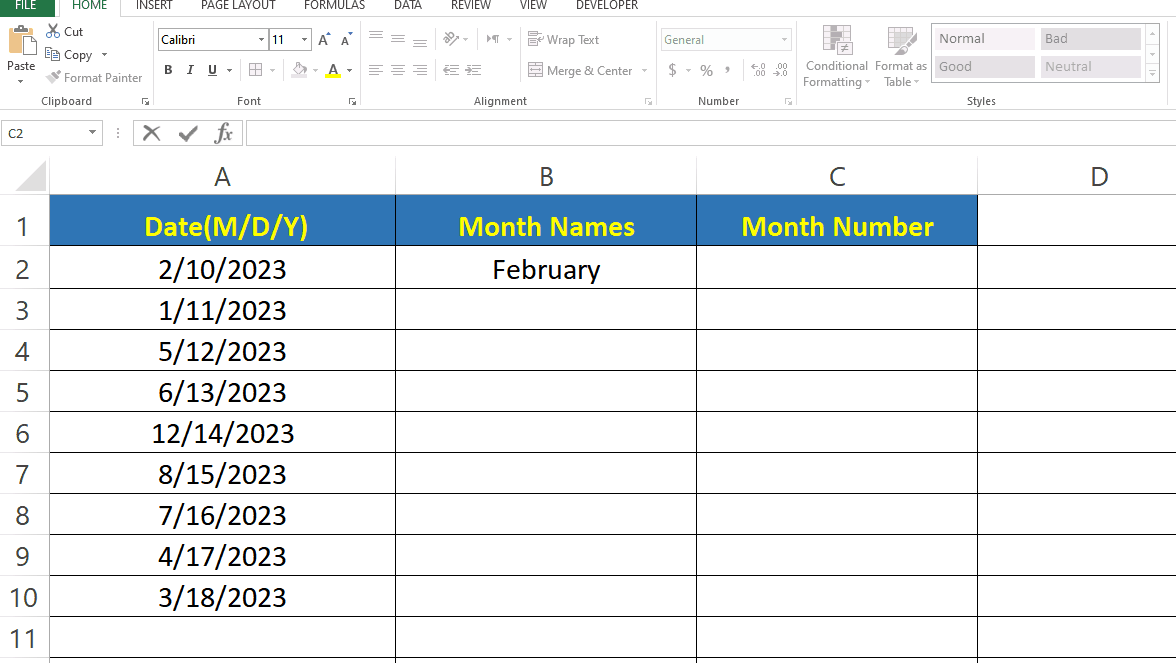# How to Extract Month from Date in Excel.If you’re working with dates in Excel, you may need to extract the month from a date at some point. Fortunately, Excel has a built-in function that makes this task simple and straightforward. Extracting the month from a date is a common task in Excel, and it can be accomplished with a simple Formula. Whether you need the Month Number or the Name of the Month, Excel provides functions that can help you extract the information you need. In this tutorial we learn how to extract Month from Date. Here we have a dataset which contains 9 random dates and we will extract Month Number and Month name from it.

### Step 1 – Extracting Month Name.– For extracting the month name from a date you have to type the formula.
– Syntax of the formula is
– In our case the cell address of date is A2.

### Step 2 – Extracting Month Number– For extracting the month number from a date you have to type the formula.
– Syntax of the formula is